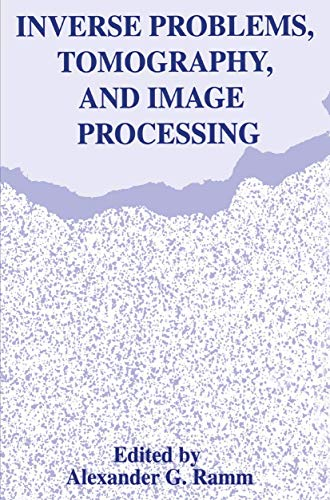• Free Shipping on all orders in Australia
• Over 7 million books in stock
• Proud to be B-Corp
• We aim to be carbon neutral by 2022
• Over 120,000 Trustpilot reviews
Item 1 of 0# Inverse Problems, Tomography, and Image Processing by Alexander G. Ramm

Condition - New
\$212.69
Only 2 left

## Summary

Proceedings of Sessions from the First Congress of the International Society for Analysis, Applications, and Computind held in Newark, Delaware, June 2-6, 1997

This book will be specially printed for your order, which means it may take a little longer to arrive (5 days). We do this to cut down on waste & to help protect our planet.

## Inverse Problems, Tomography, and Image Processing Summary

### Inverse Problems, Tomography, and Image Processing by Alexander G. Ramm

In this volume selected papers delivered at the special sessions on "Inverse problems" and "Tomography and image processing" are published. These sessions were organized by A. G. Ramm at the first international congress ofISAAC (International Society for Analysis, Appli- cations and Computing) which was held at the University of Delaware, June 3-7, 1997. The papers in this volume deal with a wide variety of problems including some theoretical and nu- merical problems arising in various inverse problems of interest in applications (Athanasiadis, Ramm and Stratis, Crosta, Gutman, Kamimura, Kochikov, Kuramshina and Yagola, Ramm, Yakhno, Yamamoto), in tomography (Faridani, Katsevich, Sharafutdinov), and in image pro- cessing (Lina, Schempp, Lobel, Pichot, Feraud and Barlaud, Weaver) These papers will be of interest to a wide audience including mathematicians, physicists, persons working in medical imaging, and theoretically oriented engineers. A.G.Ramm Manhattan, KS v CONTENTS 1. Inverse Acoustic Scattering by a Layered Obstacle C. Athanasiadis, A. G. Rarnrn, and I. G. Stratis 2. Scalar and Vector Backpropagation Applied to Shape Identification from Experimental Data: Recent Results and Open Problems ...9 Giovanni F. Crosta 3. Sampling in Parallel-Beam Tomography 33 Adel Faridani 4. Multidimensional Inverse Scattering Problem with Non-Reflecting Boundary Conditions ...55 Semion Gutman 5. Local Tomography with Nonsmooth Attenuation II 73 Alexander Katsevich 6. Inverse Problems of Determining Nonlinear Terms in Ordinary Differential Equations ...87 ...Yutaka Kamimura 7. Complex Daubechies Wavelets: Filters Design and Applications 95 J.-M. Lina 8. Edge-Preserving Regularization for Quantitative Reconstruction Algorithms in Microwave Imaging ...113 ...

Volume One:- 1: Introduction. 1.1. Why are inverse problems interesting and practically important? 1.2. Examples of inverse problems. 1.3. Ill-posed problems. 1.4. Examples of Ill-posed problems. 2: Methods of solving ill-posed problems. 2.1. Variational regularization. 2.2. Quasisolutions, quasinversion, and Backus-Gilbert method. 2.3. Iterative methods. 2.4. Dynamical system method (DSM). 2.5. Examples of solutions of ill-posed problems. 2.6. Projection methods for ill-posed problems. 3: One-dimensional inverse scattering and spectral problems. 3.1. Introduction. 3.2. Property C for ODE. 3.3. Inverse problem with I-function as the data. 3.4. lnverse spectral problem. 3.5. Inverse scattering on half-line. 3.6. Inverse scattering problem with fixed-energy. 3.7. Inverse scattering with 'incomplete data'. 3.8. Recovery of quarkonium systems. 3.9. Krein's method in inverse scattering. 3.10. Inverse problems for the heat and wave equations. 3.11. Inverse problem for an inhomogeneous Schroedinger equation. 3.12. An inverse problem of ocean acoustics. 3.13. Theory of ground-penetrating radars. Volume Two:- 4: Inverse obstacle scattering. 4.1. Statement of the problem. 4.2. Inverse obstacle scattering problems. 4.3. Stability estimates for the solution to IOSP. 4.4. High-frequency asymptotics. 4.5. Remarks about numerical methods. 4.6. Analysis of a method for identification ofobstacles. 5: Inverse scattering problem. 5.1. Introduction. 5.2. Inverse potential scattering problem with fixed-energy data. 5.3. Inverse geophysical scattering with fixed-frequency data. 5.4. Proofs of some estimates. 5.5. Construction of the Dirichlet-to-Neumann map. 5.6. Property C. 5.7. Necessary and sufficient condition for scatterers. 5.8. The Born inversion. 5.9. Uniqueness theorems for inverse spectral problems. 6: Non-uniqueness and uniqueness results. 6.1. Examples of nonuniqueness for an inverse problem of geophysics. 6.2. A uniqueness theorem for an inverse problem. 6.3. Property C and an inverse problem for a hyperbolic equation. 6.4. Continuation of the data. 7: Inverse problems of potential theory. 7.1. Inverse problem of potential theory. 7.2. Antenna synthesis problems. 7.3. Inverse source problem for hyperbolic equations. 8: Non-overdetermined inverse problems. 8.1. Introduction. 8.2. Assumptions. 8.3. The problem and the result. 8.4. Finding lpj(s) from lp](s). 8.5. Appendix. 9: Low-frequency inversion. 9.1. Derivation of the basic equation. Uniqueness results. 9.2. Analytical solution of the basic equation. 9.3. Characterization of the low-frequency data. 9.4. Problems of numerical implementation. 9.5. Half-spaces with different properties. 9.6. Inversion of the data given on a sphere. 9.7. Inversion of the data given on a cylinder. 9.8. Two-dimensional inverse problems. 9.9.

NLS9781489919007
9781489919007
1489919007
Inverse Problems, Tomography, and Image Processing by Alexander G. Ramm
New
Paperback
Springer-Verlag New York Inc.
2013-06-22
258
N/A
Book picture is for illustrative purposes only, actual binding, cover or edition may vary.
This is a new book - be the first to read this copy. With untouched pages and a perfect binding, your brand new copy is ready to be opened for the first time